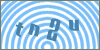Products
ServicesScripts: asp :: Math Functions :: Library Article #15Developer's SectionRound a Decimal Number Up using ASP (Math.Ceil Function Equivalent)
By: Erobo Team Member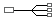Hire a Developer for Related Work / Installation | \$55 hr
 Rating: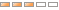| Rate It: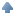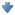Average Votes: (1932) Favorites:In this tutorial we will be explaining how to create a Math.Ceil Function using the available functions on ASP.

You can round a number up on classic asp by using a function that computes the integer of itself and adds to that the result of computing the asp Sgn() of the decimal number minus the int value of that number. Thus, the Sgn() function either adds 1 to round it to the next number or returns 0 if it is equal.

See Example below: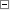Code Snippet 1<%
'our round up function
Function RoundUp(val)
RoundUp = Int(val) + Sgn(val - Int(val))
End Function

'compute the number of batches based off the round up calculation
Dim arrayOfRecipients
arrayOfRecipients = Split(Request("recipients"),",")

Dim batchCapacity : batchCapacity = 5

Dim numberOfBatches : numberOfBatches = 0

if UBound(arrayOfRecipients) < batchCapacity then
numberOfBatches = 1
else
numberOfBatches = Clng(RoundUp(UBound(arrayOfRecipients) / batchCapacity))
end if

%>See other Scripts in Math FunctionsSubmit Your Scripts:If you would like to have your ASP & ASP.NET scripts published in this section please fill outthe form below:
 [ Refresh Image ]